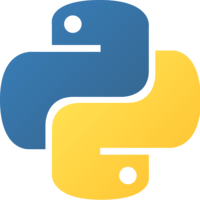Unlock This Lesson
1×
Become a member
to unlock all featuresLevel Up!

Access all courses & lessons on egghead today and lock-in your price for life.

Autoplay

# Use Comparison Operators in Pythonpython
3

Use comparison operators such as >, <, >=, <=, ==, and != to determine the next actions taken by your Python application. You will also learn how to identify if your object is a specific type, such as integer or string.### Code### You must be a Member to view code

Access all courses and lessons, track your progress, gain confidence and expertise.

Become a Member
and unlock code for this lesson### Transcript

In python, we can use the double equals sign to test for equality. 5=5, it returns true, or 5==4 will return false. We can use the exclamation point and equals sign to test for inequality, so 5!=4. We can use the greater than operator to say 5>3, and less than operator to say 3<5.

You can combine the greater than and less than symbols with the equals sign to test for, say, 5>=3, or 5>=5, and that still returns true. It works with objects, too. If we have a list with the values 1, 2, and 4, and that's greater than 1, a list containing 1, 2, and 3, that will return true as well.

We can use the Boolean operators to say 1<2 and 5>4. That returns true. Because the Boolean operators operate at a lower priority than the other comparison operators, it's equivalent to writing it out this way, with (1<2) in parenthesis, and (5>4) in parenthesis.

Parenthesis can specify the order of operation as well, but because the Booleans operate at a lower priority, it's the same without the parenthesis. You can do 1>2 or 5>4, and that will return true using the or Boolean operator.

Operators can be chained together also. If we have x=4, we can write x>3 and x<5, and that returns true. Another way to write this with chaining would be 3<x<5, and that's the same thing.

Comparing data types can be done with the easy instance method. If we have a string called Will, when comparing it to a string, it'll return true. If we compared it to an integer, it would return false. If we compare the value 4.0 against a float, that returns true as well.

The is comparison operator checks to see if it's the exact same object. If we had a=true, and b=true, we can say a is b, and that returns true. Look at this. If we have x, which is a list containing the values 1, 2, and 3, and we have a variable, y, which is a list containing the values 1, 2, and 3, when I type x is y, that returns false. Let me show you why.

In the original example, with a and b, if we take a look at the ID of those, the ID is the exact same value, so is returns true, but the IDs for x and y are different values, meaning that they are different objects within Python, so it returns false.

The in comparison operator, on the other hand, checks the value of the object. X is an array with the values 1, 2, and 3. If I type 3 in x, that returns true. If I type 5 in x, that returns false, because it looks for the value that's being compared in the object, not the ID object itself.

Using the comparison operators like these as standalone statements is helping in returning when they evaluate to true, or when they evaluate to false. Most commonly, what you're going to do is you're going to use it in combination with the flow control statement like if, while, or for to create the logic for your applications.

We have x, which is our list containing the values 1, 2, and 3. For the value in x, if the value is equal to 2, we'll print the value as 2. What happens there is the for starts a loop that iterates through each item in our list contained within x. It evaluates the value. If that value is equal to 2, then it executes the code found within the print block which, for us, is printing out value is 2.

Let me show you another example with a dictionary, or a dict. We have the dictionary, car, that has three keys in it, model, year, and color. Start an if statement, and say if model and car, we'll print out this is a, and include the positional operator with the format method, and then from the dictionary, grab the value of the key model from the car dictionary.

When that runs, it does a lookup of the keys in the dictionary. If it finds a key named model, then it prints out the statement with the value that it found for that key.